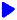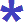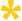Noetic Learning Math Worksheet Creator

Generate Number Sense WorksheetsBack to Worksheet Creator HomeCompare numbers

Numbers are no more than

Number of questions:   questions per row: Generate!Write numbers in standard form

Numbers are no more than

Number of questions: questions per row:  Generate!Place value     ( ex. what digit is in 3,245 tens place? )

Numbers are no more than

Number of questions: questions per row:  Generate!Place value 2  ( ex. what place is 4 in 3,245? )     free sample

Numbers are no more than

Number of questions: questions per row:  Generate!Rounding     free sample

Numbers are no more than

Number of questions: questions per row:  Generate!